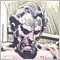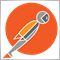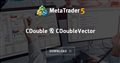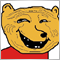# Rounding but not approximation [Code help]894

Hello all:) I've been working on several systems and I want to implement a function that would require a rounding process but not approximation, so far I've been using normalize double but I can't reach my goal with this function. Any ideas?

Example:

Turning 5.19 into 5.1 (Not rounding to the nearest value)

With the NormalizeDouble I do get my goal of transforming 5.19408523245 (this is a random number) into a 1 decimal value,but instead of making it 5.1 it rounds up and then turning the actual value to 5.2

Regards,

Fernando.3197

Turning 5.19 into 5.1 (Not rounding to the nearest value)
```void OnStart()
{
double numberTest = 5.19;

double truncated = ((long) (numberTest * 10) / 10.);
PrintFormat("%f", truncated);
}

```19130

Anthony Garot:
Don't write one time use code. Write generalized functions.
MT4:NormalizeDouble - General - MQL5 programming forum
How to Normalize - Expert Advisors and Automated Trading - MQL5 programming forum
Fernando Jose Velasco Borea: With the NormalizeDouble

See links provide. Never use NormalizeDouble.3197

whroeder1:
Don't write one time use code. Write generalized functions.

Knowing how to truncate a number is more informative than being handed a general function.2380

The recommended method is to round down.

```double roundToStepDown(double number, double step)
{
return step * floor(number / step);
}```

So in this case:

`double num = roundToStepDown(5.19, 0.1);`

Also, the easiest way is to use the already existing CDouble library. It has all the methods you need for working with floating point numbers.

https://www.mql5.com/en/code/19727CDouble & CDoubleVector
• www.mql5.com
A library for common rounding methods used in MQL development, primitive wrapper class for type (double), and vector for CDouble objects. MQL5 and MQL4 compatible! CDouble The CDouble class wraps a value of the primitive type double in an object. Additionally, this class provides several methods and static methods for rounding doubles and...1084

nicholi shen:

```double roundToStepDown(double number, double step)
{
return step * floor(number / step);
}```

```double roundToStepDown(double number, double step)
{
if(number>=0) return step * floor(number / step);
else return step * ceil(number / step);
}```3197

kypa:
Good catch. It appears the floor() function rounds towards negative infinity, and isn't suited for negative numbers.1084

It should round up to the bigger digit. If it rounds up to a much bigger digit the problem might be double/float precision, not really the function.

I don't really know though, I don't understand these quite well.3197

whroeder1:
Don't write one time use code. Write generalized functions.

The thing about @whroeder1 is that he pushes me to not be lazy.

Here's my solution as a generalized function.

```// Truncates positive or negative numbers to the specified precision
double MathTruncate(const double number, const uint digits)
{
double multiplier = MathPow(10,digits);
return (long) (number * multiplier) / multiplier;
}```
Per https://en.wikipedia.org/wiki/Truncation#Truncation_and_floor_function